Courses

# Carnot Cycle Class 11 Notes | EduRev

## Class 11 : Carnot Cycle Class 11 Notes | EduRev

The document Carnot Cycle Class 11 Notes | EduRev is a part of the Class 11 Course Chemistry Class 11.
All you need of Class 11 at this link: Class 11

CARNOT CYCLE
Carnot cycle is based on 4 reversible process.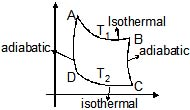(1) Reversible isothermal expansion from A to B.
ΔEAB = 0 ,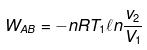(2) Reversible adiabatic expansion from B to C
ΔEBC = nCV(T2 -T1)
WBC = ΔEBC

(3) Isothermal compression from C to D
ΔECD = 0,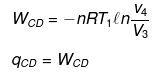(4) Adiabatic compression from D to A.
ΔEDA  = nCV(T- T2)
WDA = ΔEDA
ΔECycle  = 0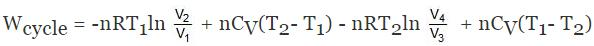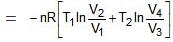For BC,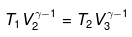For ΔA,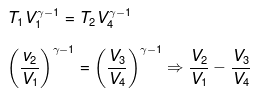Wcycle = -nR(T1 - T2)ln V2/V1

Efficiency of any engine may be given as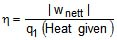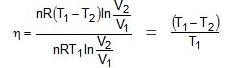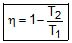ΔEcycle = qcyc wcycle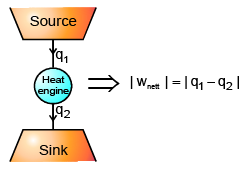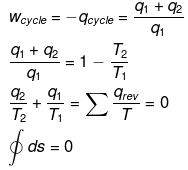This means ΔS is a state function

Gibb's Free Energy (G)
Gsystem = Hsystem - TSsystem
W = Wexpansion +Wnon-expansion
Wnon - expansion = wuseful (useful work)
ΔG = Wnon expansion = Wuseful
All those energy which is available with the system which is utilized in doing useful work is called Gibb's free energy :

Rx
1. ΔGsystem ΔHsystem - TΔSsystem = - TΔSuniverse = Wnon expansion = Wuseful
2. ΔGsystem = - TΔSuniverse
3.
(a) ΔSuniverse > 0 or ΔGsystem < 0 ⇒ Spontaneous
(b) ΔSuniverse = 0 or ΔGsystem = 0 ⇒ Equilibrium
(c) ΔSuniverse < 0 or ΔGsystem > 0 ⇒ Non-Spontaneous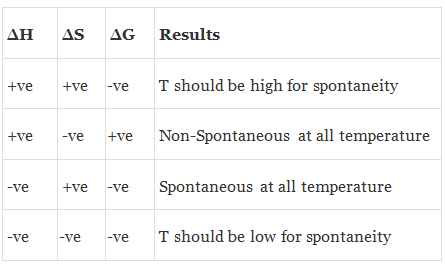APPLICATION OF ( ΔG )
Rx
(1) aA + bB  ⇌ cC + dD
ΔG° = Standard Gibb's free energy change (P = 1 atm, 298 K)
ΔG = Gibb's free energy change at any condition.

NERNST EQUATION
ΔG =  ΔG° + 2.303 RT log Q ; Q = Reaction Quotient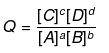At equilibrium, ΔG = 0 and Q = Keq.
0 = ΔG° 2.303 RT log keq
ΔG° = -2.303 RT log keq
å G°(product) - å G° (Reactant) = - 2.303 RT log keq
ΔH° - T ΔS° = -2.303 RT log keq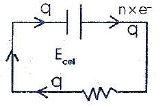(2) Wcell = q × E
ΔG = - Wcell
ΔG = - q × Ecell
Now, one mole e- have charge 96500 coulomb = 1 Faraday (F)
n mole of e- will have charge = n × F or q = n × F
ΔG = - nFEcell
ΔG° = -nFE°cell

THIRD LAW OF THERMODYNAMICS
limitT→0 S = 0
Third law of thermodynamics states that as the temperature approaches absolute zero, the entropy of perfectly crystalline substance also approaches zero.

APPLICATION OF THIRD LAW OF THERMODYNAMICS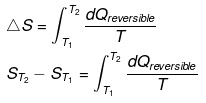Taking T2 = T and T1 = 0°k.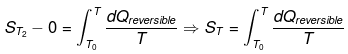For perfectly crystalline substance. The entropy of perfectly crystalline substance can be determined using third law of thermodynamics.
or
With the help of third law of thermodynamics we can calculate the exact value of entropy.

ΔG AND NON PV WORK (NON EXPANSION/COMPRESSION)
(ΔG)T,P  is a measure of useful work a non PV work (non expansion work) that can be produced by a chemical transformation.e.g.electrical work .

For reversible reaction at constant T & P
dU = dq + dWtotal
dU = dq + dWP, V + dWnon P,V
dU = T.dS - P. dV + dWnon P,V
dU + P.dV = T. dS + dWnon P,V
dH = T.dS + dWnon P,V
(dGsys)T,P = dWnon P,V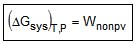Useful work done on the system = increase in Gibb's energy of system at constant T & P.
- (ΔGsys)T,P= - Wnon P,V
- (ΔGsys)T,P= - Wby, non P,V
Useful work done by the system = decrease in Gibb's energy of system at constant T & P.
If (ΔGsys)T,P = 0, then system is unable to deliver useful work.

THERMODYNAMIC RELATION
For reversible process in which non-expansion work is not possible
dU = dq + dW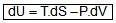H = U + PV
dH = dU + P.dV + VdP
dH = T.dS - PdV + PdV + V.dP
dH = T.dS + V.dP
G = H -TS
dG = dH - T.dS -S.dT
dG = T.dS + V.dP - T.dS - S.dT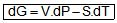For a particular system (s/ℓ/g)

(1) At constant temperature dG = V.dP
or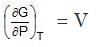(A) For a system is s/ ℓ phase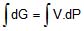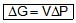(B) For an ideal gas, expansion/compression:-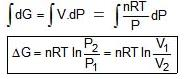(2) At constant pressure : dG =-S.dT
or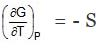For phase transformation/chemical reaction
d(ΔG) = ΔV.dP - ΔS dT
H2O(s) → H2O(l)
ΔV = VM(H2O,l) - Vm(H2O,s)
ΔS = SM(H2O,l) - Sm(H2O,s)
A(s) → B(g) + 2C(g)
ΔrS = SM(B,g ) 2Sm(C,g) - Sm(A,s)
C (s, graphite) → C(s, diamond)
ΔV = Vm(C, diamond) - Vm(C-graphite)
At constant temperature:-
d(ΔG) = ΔV. dP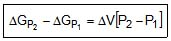At constant pressure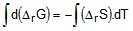ΔrGT2 - ΔrGT1 = - ΔrS(T2 - T1)

Offer running on EduRev: Apply code STAYHOME200 to get INR 200 off on our premium plan EduRev Infinity!

## Chemistry Class 11

136 videos|245 docs|180 tests

,

,

,

,

,

,

,

,

,

,

,

,

,

,

,

,

,

,

,

,

,

;